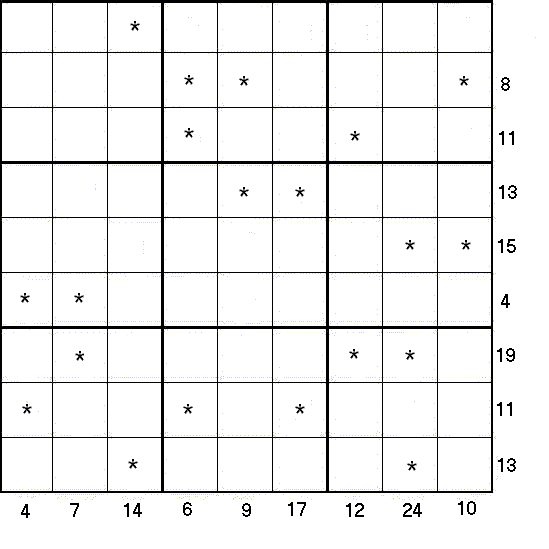#### You may also like### Not a Polite Question

When asked how old she was, the teacher replied: My age in years is not prime but odd and when reversed and added to my age you have a perfect square...### Whole Numbers Only

Can you work out how many of each kind of pencil this student bought?### Symmetricality

Add up all 5 equations given below. What do you notice? Solve the system and find the values of a, b, c , d and e. b + c + d + e = 4 a + c + d + e = 5 a + b + d + e = 1 a + b + c + e = 2 a + b + c + d = 0

#### By Henry Kwok### Rules of Addition Equation Sudoku

Like the standard sudoku, this sudoku has the basic rule:
• Every row, every column and every 3x3 box in the grids contains the digits 1 through 9.
The puzzle can be solved by finding the values of the unknown digits (all indicated by asterisks) in the squares of the 9x9 grid. At the bottom and right side of the 9x9 grid are numbers, each of which is the sum of a column or row of unknown digits marked by asterisks.

In this puzzle, a set of 17 equations can be formed from the columns and rows of unknown digits and constants. For example, in the fourth and fifth columns beginning from the left of the 9x9 grid, the following equations can be formed:
$* + * + * = 6$ and
$* + * = 9$.

In the eighth and ninth rows beginning from the top of the 9x9 grid, the following equations can be formed:
$* + * + * = 11$ and
$* + * = 13$.

After solving all the equations, the puzzle is solved by the usual sudoku technique and strategy.

A printable version of the problem can be found here.